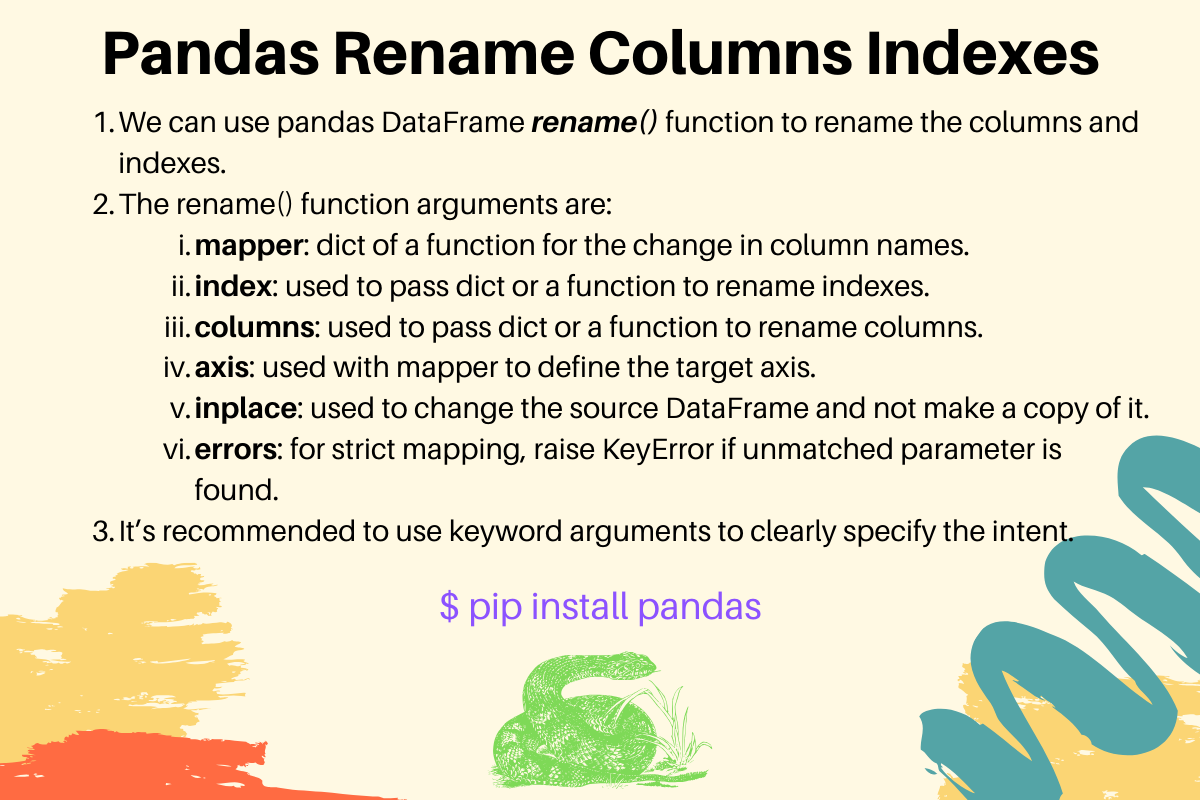# Pandas Rename Column and Index

Published on August 3, 2022By PankajWhile we believe that this content benefits our community, we have not yet thoroughly reviewed it. If you have any suggestions for improvements, please let us know by clicking the “report an issue“ button at the bottom of the tutorial.

Sometimes we want to rename columns and indexes in the Pandas DataFrame object. We can use pandas DataFrame rename() function to rename columns and indexes. It supports the following parameters.

• mapper: dictionary or a function to apply on the columns and indexes. The ‘axis’ parameter determines the target axis - columns or indexes.
• index: must be a dictionary or function to change the index names.
• columns: must be a dictionary or function to change the column names.
• axis: can be int or string. It’s used with ‘mapper’ parameter to define the target axis. The allowed values are (‘index’, ‘columns’) or number (0, 1). The default value is ‘index’.
• inplace: if True, the DataFrame is changed. Otherwise, a new DataFrame is returned and the current DataFrame remains unchanged. The default value is ‘False’.
• level: can be int or level name. It’s used in case of a MultiIndex, only rename labels in the specified level.
• errors: possible values are (‘ignore’, ‘raise’), default is ‘ignore’. If specified as ‘raise’ then KeyError is raised when a dict-like ‘mapper’, ‘index’, or ‘columns’ contains labels that are not present in the Index being transformed. If ‘ignore’, existing keys will be renamed and extra keys will be ignored.

Some important points about rename() function.

1. It’s recommended to use keyword arguments to clearly specify the intent.
2. We can rename single column or multiple columns with this function, depending on the values in the dictionary.

Let’s look into some examples of using Pandas rename() function.

## 1. Pandas Rename Columns

``````import pandas as pd

d1 = {'Name': ['Pankaj', 'Lisa', 'David'], 'ID': [1, 2, 3], 'Role': ['CEO', 'Editor', 'Author']}

df = pd.DataFrame(d1)

print('Source DataFrame:\n', df)

# rename columns
df1 = df.rename(columns={'Name': 'EmpName', 'ID': 'EmpID', 'Role': 'EmpRole'})
print('Result DataFrame:\n', df1)
``````

Output:

``````Source DataFrame:
Name  ID    Role
0  Pankaj   1     CEO
1    Lisa   2  Editor
2   David   3  Author
Result DataFrame:
EmpName  EmpID EmpRole
0  Pankaj      1     CEO
1    Lisa      2  Editor
2   David      3  Author
``````

The above rename() function call can also be written in the following way.

``````df1 = df.rename(mapper={'Name': 'EmpName', 'ID': 'EmpID', 'Role': 'EmpRole'},
axis='columns')  # axis=1 corresponds to columns
``````

It’s clear that using the keyword arguments is clearer than using the mapper and axis arguments.

## 2. Pandas Rename Single Column

If you want to rename a single column, just pass the single key-value pair in the columns dict parameter.

``````df1 = df.rename(columns={'Name': 'EmpName'})
print(df1)
``````

Output:

``````  EmpName  ID    Role
0  Pankaj   1     CEO
1    Lisa   2  Editor
2   David   3  Author
``````

The result will be the same if there is a non-matching mapping in the columns dictionary.

``````df1 = df.rename(columns={'Name': 'EmpName', 'X': 'Y'})  # same result since there is no X column
``````

## 3. Pandas Rename Indexes

If you want to rename indexes, pass the dict for ‘index’ parameter.

``````df2 = df.rename(index={0: '#0', 1: '#1', 2: '#2'})
print('Renamed Indexes:\n', df2)
``````

Output:

``````Renamed Indexes:
Name  ID    Role
#0  Pankaj   1     CEO
#1    Lisa   2  Editor
#2   David   3  Author
``````

We can also rename indexes using mapper and axis arguments.

``````df2 = df.rename({0: '#0', 1: '#1', 2: '#2'}, axis=0)
# axis='index' will work, first argument is assigned to 'mapper'
``````

## 4. Pandas Rename Single Index

``````df2 = df.rename(index={1: '#1'})
print(df2)
``````

Output:

``````      Name  ID    Role
0   Pankaj   1     CEO
#1    Lisa   2  Editor
2    David   3  Author
``````

## 5. Changing the DataFrame inplace

If you want to change the source DataFrame itself, pass the inplace argument as True.

``````import pandas as pd

d1 = {'Name': ['Pankaj', 'Lisa', 'David'], 'ID': [1, 2, 3], 'Role': ['CEO', 'Editor', 'Author']}

df = pd.DataFrame(d1)

print('Source DataFrame:\n', df)

df.rename(index={0: '#0', 1: '#1', 2: '#2'}, columns={'Name': 'EmpName', 'ID': 'EmpID', 'Role': 'EmpRole'}, inplace=True)

print('Source DataFrame:\n', df)
``````

Output:

``````Source DataFrame:
Name  ID    Role
0  Pankaj   1     CEO
1    Lisa   2  Editor
2   David   3  Author

Source DataFrame:
EmpName  EmpID EmpRole
#0  Pankaj      1     CEO
#1    Lisa      2  Editor
#2   David      3  Author
``````

## 6. Using mapper function to rename columns

``````df = pd.DataFrame({'NAME': ['Pankaj', 'Lisa'], 'ID': [1, 2], 'ROLE': ['CEO', 'Editor']})
print(df)

df.rename(mapper=str.lower, axis=1, inplace=True)
print(df)
``````

Output:

``````     NAME  ID    ROLE
0  Pankaj   1     CEO
1    Lisa   2  Editor

name  id    role
0  Pankaj   1     CEO
1    Lisa   2  Editor
``````

## 7. Using functions to rename columns and indexes

``````import pandas as pd
import math

df = pd.DataFrame({'NAME': ['Pankaj', 'Lisa'], 'ID': [1, 2], 'ROLE': ['CEO', 'Editor']})

df.rename(columns=str.lower, index=math.degrees, inplace=True)
print(df)
``````

Output:

``````            name  id    role
0.00000   Pankaj   1     CEO
57.29578    Lisa   2  Editor
``````

## 8. Strict Rename and Raising KeyError

``````import pandas as pd

df = pd.DataFrame({'NAME': ['Pankaj', 'Lisa'], 'ID': [1, 2], 'ROLE': ['CEO', 'Editor']})

df1 = df.rename(columns={'Salary': 'EmpSalary'})  # unmatched mappings are ignored

df1 = df.rename(columns={'Salary': 'EmpSalary'}, errors='raise')  # unmatched mappings raising KeyError
``````

Output:

``````Traceback (most recent call last):
File "/Users/pankaj/Documents/PycharmProjects/hello-world/journaldev/pandas/pandas_rename_column.py", line 58, in <module>
df1 = df.rename(columns={'Salary': 'EmpSalary'}, errors='raise')
``````

## 9. References

Thanks for learning with the DigitalOcean Community. Check out our offerings for compute, storage, networking, and managed databases.Pankaj

author

#### Still looking for an answer?

Ask a questionSearch for more help

Click below to sign up and get \$200 of credit to try our products over 60 days!

### Popular Topics

##### Join the Tech Talk##### Hollie's Hub for Good

Working on improving health and education, reducing inequality, and spurring economic growth? We’d like to help.